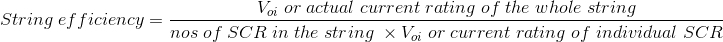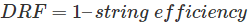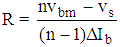# Series and Parallel Connection of SCR or Thyristor

Nowadays, SCRs are available of ratings up to 10 KV and 3 KA. But sometimes we face demand, more than these ratings. In this case combination of more than one SCRs is used. Series connection of SCRs meets high voltage demand and parallel connection of SCRs meets high current demand. These series and parallel connection of SCR or Thyristor will work efficiently if all SCRs are fully utilized. Although all SCRs in a string are of same rating, their V-I characteristics differ from one another. This leads to unequal voltage or current division among them. Hence every SCR is not fully utilized. So the efficiency of string is always less than 100% according to the given expressionWith increase in the numbers of SCRs in a string voltage or current handled by each SCR is minimized. This phenomenon increases the reliability of the string, but reduces the utilization of each SCR. Thus string efficiency decreases. Reliability of string is measured by derating factor (DRF) which is given by the expression## Series Operation of SCR

When the operating voltage is more than the rating of one SCR the multiple SCRs of same ratings are used in series. As we know SCR’s having same rating, may have different I-V characteristic, so unequal voltage division is bound to take place. For example if two SCRs in series that is capable of blocking 5 KV individually, then the string should block 10 KV. But practically this does not happen. This can be verified with the help of an example. Let the characteristics of two SCRs are as shown in fig. 1.

So we can see from the diagram, for same leakage current, unequal voltage division takes place. Voltage across SCR1 is V1 but that across SCR2 is V2. V2 is much less than V1. So, SCR2 is not fully utilized. Hence the string can block V1 + V2 = 8 KV, rather than 10 KV and the string efficiency is given by = 80%.

To improve the efficiency a resistor in parallel with every SCR is used. The value of these resistances are such that the equivalent resistance of each SCR and resistor pair will be same. Hence this will ensure equal voltage division across each SCR. But in practical different rating of resistor is very difficult to use. So we chose one value of resistance to get optimum result which is given byWhere, n = no. of SCR in the string
Vbm = Voltage blocked by the SCR having minimum leakage current.
ΔIb = Difference between maximum and minimum leakage current flowing through SCRs.
Vs = Voltage across the string.
This resistance b is called static equalizing circuit. But this resistance is not enough to equalize the voltage division during turn on and turn off. In these transient conditions, to maintain the equal volume across each device a capacitor is used along with resistor in parallel with every SCR. This is nothing but snubber ckt which also known as dynamic equalizing circuit. An additional diodes can also be used to improve the performance of dynamic equalizing circuit.

## Parallel Operation of SCR

When the operating current is more than the individual current ratings of SCRs then we use more than one SCRs in parallel. Due to different V-I characteristics SCRs of same rating shares unequal current in a string. Let a string consists of two transistors in parallel as shown in fig. 1 and their current rating by 1 KA. From the V-I characteristics of the devices it can be seen that for operating volume V, current through SCR1 is 1 KA and that through SCR2 is 0.8 KA. Hence, SCR2 is not fully utilized here. Though the string should withstand R KA theoretically it is only capable of handling 1.8 KA. So, the string efficiency is = 90%.

Due to unequal current division when current through SCR increases, its temperature also increases which in turn decreases the resistance. Hence further increase in current takes place and this is a cumulative process. This is known as thermal ‘run away’ which can damage the device. To overcome this problem SCRs would be maintained at the same temperature. This is possible by mounting them on same heat sink. They should be mounted in symmetrical position as flux.

Linkages by the devices will be same. So, the mutual inductance of devices will be same. This will offer same reactance through every device. Thus reducing the difference in current level through the devices. Another way of equalizing the current division in ac circuit can be achieved by using magnetic coupled reactance as shown in Fig – 2.

When I1 = I2 then resultant flux is zero as two coils are connected in anti-parallel. So, the inductance of the both path will be same. If I1 > I2 then there will be a resultant flux. This flux induces emfs in cols. 1 and 2 as shown in fig. Hence current in path 1 is opposed and in path 2 it is aided by the induced emfs. Thus reducing the current difference in the paths.

Want To Learn Faster? 🎓
Get electrical articles delivered to your inbox every week.
No credit card required—it’s 100% free.